# Subtraction of One Fraction from Another

Reduce the fractions to their simplest forms, reduce the resulting fractions to their common denominator and then subtract the smaller numerator from the larger one. If the two numbers consist of whole numbers and simple fractions, again subtract the smaller from the larger. If the fraction in the subtrahend is larger than that of the minuend then borrow 1 from the whole number in the minuend.

Examples

(1) Subtract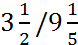from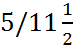.

This is calculated as: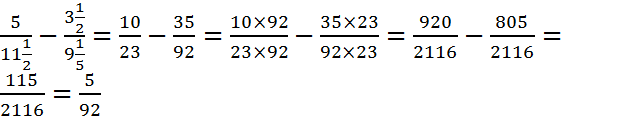(2) Subtract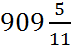from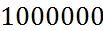.

Expressas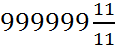. Then the difference in the whole numbers is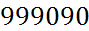and the difference in the fractions is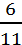so that the answer is.